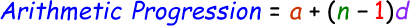# Arithmetic Progression Calculator

The arithmetic progression also called as arithmetic sequence can be explained as the sequence of numerals in such a way that the difference of any two successive numerals of the sequence is a constant.

Formula to calculate arithmetic progression can be given by:here,
a = First term in the series
n = Last term in the series
d = Common difference

In out below online arithmetic progression calculator, enter the values in the input boxes and click calculate to find the sum of arithmetic progression.

 First Term in the Series (a): Last Term in the Series, nth term (n): Common Difference (d): Arithmetic Progression:

Latest Calculator Release

Average Acceleration Calculator

Average acceleration is the object's change in speed for a specific given time period. ...

Free Fall Calculator

When an object falls into the ground due to planet's own gravitational force is known a...

Torque Calculator

Torque is nothing but a rotational force. In other words, the amount of force applied t...

Average Force Calculator

Average force can be explained as the amount of force exerted by the body moving at giv...

Angular Displacement Calculator

Angular displacement is the angle at which an object moves on a circular path. It is de...